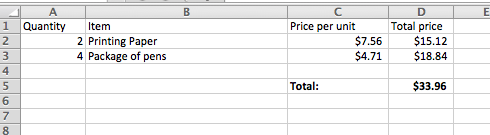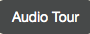# Figuring out an Invoice¶

We can use variables to solve problems like those we might solve in a spreadsheet. Imagine that you had a spreadsheet with an invoice for an office supply company.Figure 3: A spreadsheet with order information

Here’s a program to compute the total price for the invoice. Be sure to clickto understand what’s happening here.

csp-3-8-1: How many variables are in this program?

• 7
• Yes, quantity1, unitPrice1, total1, quantity2, unitPrice2, total2, invoiceTotal.
• 6
• There are three variables per line, two lines, and one total.
• 5
• There are three variables per line, two lines, and one total.
• 2
• There are three variables per line, two lines, and one total.

We don’t really have to create new variables `quantity2` and `unitPrice2`. We only use those to compute the total for the line, and then we could reuse those variable names.

CodeLens: (Invoice2)

csp-3-8-2: How many variables are in this program?

• 7
• We have two fewer variables now.
• 6
• We have a total for each line (two), a quantity, a unitPrice, and an invoiceTotal.
• 5
• The variables are quantity, unitPrice, total1, total2, and invoiceTotal.
• 2
• We have a total for each line (two), a quantity, a unitPrice, and an invoiceTotal.

Note

It is best to use variable names that make sense like `invoiceTotal` and `quantity` instead of names that don’t make any sense like `thisVariableIsMyFriend` and `Fred`. The name should help you remember what the variable is representing.

Let’s say that apples are \$0.40 apiece, and pears are \$0.65 apiece. Modify the program below to calculate the total cost.

You are welcome to try out the following answers by copying and pasting them into the program above before answering this question:

csp-3-8-3: Which line of code will compute the correct `totalCost` if put into line 3 above?

• totalCost = apples + pears
• That does not consider the cost of the apples or pears.
• totalCost = (0.4 * apples) + (0.65 * pears)
• We need to multiply the cost per apple times the number of apples and add it to the cost per pear times the number of pears.
• totalCost = (0.4 * pears) + (0.65 * apples)
• That gets the costs backwards
• totalCost = (0.4 + apples) * (0.65 + pears)
• That is the wrong formula for computing total cost.

Write the code to calculate and print how many paperclips you can buy if each paperclip is \$0.05 and you have \$4.00 in your pocket. It should print 80.

Create variables to hold each value. Calculate `numPaperclips` as `budget / costPerClip`. Be sure to print the result.